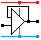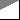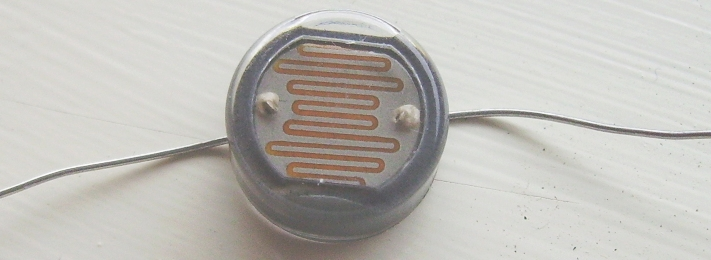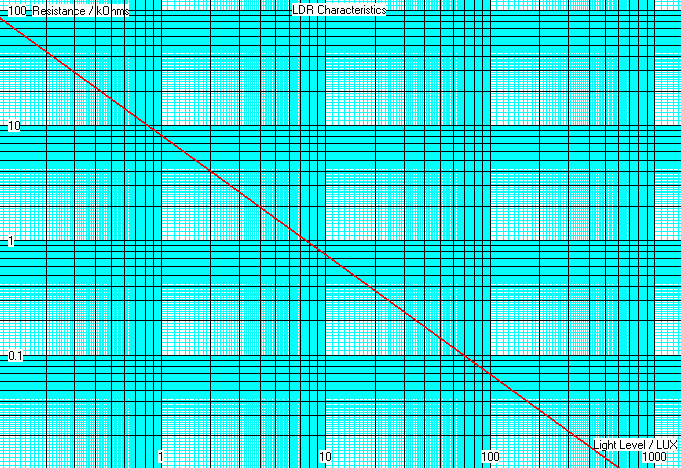Home

rOm
Quest
Glossary

Random
Page
Search
Site
Lush
Sim
Class
Subject
Images

Help
FAQ
Sign
Up
Log
In
 GCSE     Resistors     BS1852     Colour Codes     E24     >LDR<     Parallel     PIVR Calculations     Potentiometer     Puzzles     Series     Thermistor     Timing     Types     Voltage Divider

# Resistors LDR

Site for Eduqas/WJEC - Go to the AQA site.

GCSE    ResistorsQuestions 0 to 27   -->  View All

The LDR is an analogue, passive, resistive, input transducer used to sense light intensity.## Light Dependent Resistor Properties

• The LDR Resistance decreases with increasing light intensity.
• LDRs are used to measure the light level.
• They are slow to react so they are not used with fibre-optic or other optical communications systems.
• They are used in light meters for photography and for systems that turn on lights when it gets dark.
• The LDR is normally used with a fixed resistor. This prevents too much current flowing when the light is very bright.
• The LDR and fixed resistor convert the varying light level into a varying voltage.

## LDR Protection

The LDR is wired up with a resistor in series.
This makes a voltage divider circuit.
The series resistor is chosen to satisfy two
design constraints.

• When the light is bright and the LDR
resistance drops close to zero, the other
resistor MUST ensure that the LDR
current remains below a safe limit.
• It is also desirable if the LDR
resistance and the series resistance
are approximately equal at the light
level you are trying to detect.

### Calculate the Safety Resistor

Assume the LDR resistance is small in very
bright light. In a 12 Volt circuit, if the maximum
safe current is 10 mA ...

R = V / I      R = 12 / 0.01      R = 1.2KΩ

## Voltage DividerVout = 12 x R2 / ( R1 + R2 )

As the light gets brighter, R2
decreases and the output
voltage drops.

## Voltage DividerVout = 12 x R2 / ( R1 + R2 )

As the light gets brighter, R1
decreases and the output
voltage rises.

## How the LDR Works

Light Dependent Resistors (LDR) are made from a semiconductor. In the dark, this semiconductor has very few free carriers so the LDR has a high resistance. When photons of light hit the semiconductor, they add enough energy to dislodge carriers which can then flow. This reduces the resistance. The carriers are electrons.

## Logarithmic Graphs

As the light level changes, the resistance of the LDR changes over a huge range. This is difficult to plot onto normal graph paper so logarithmic graphs are used.• If the resistance is 20kΩ, the light level is about 0.42 Lux.
• If the resistance is 2kΩ, the light level is 4 Lux.
• If the light level is 10 Lux, the resistance is 750Ω.
• If the light level is 600 Lux, the resistance is about 10Ω which is getting close to zero.

## Calculating the Safety Resistor

• For this LDR, the absolute maximum current is 50mA.
• In bright light, assume the LDR resistance drops to zero.

If the LDR is in a 12 volt circuit, the series resistor must be at least ...

R = V / I

R = 12 / 50x10-3

R = 240Ω

240Ω is the minimum safe resistance.

If the goal is to detect a light level less than 10 Lux, a more suitable resistor would be about 800Ω.

This resistor satisfies both the design requirements mentioned at the top of this page.

At what light level does it become impossible to meet both design requirements?

Subject Name     Level     Topic Name     Question Heading     First Name Last Name Class ID     User ID

 CloseEscapeX Q: qNum of last_q     Q ID: Question ID         Score: num correct/num attempts         Date Done

Question Text

image url

Help Text
Debug

• You can attempt a question as many times as you like.
• If you are logged in, your first attempt, each day, is logged.
• To improve your scores, come back on future days, log in and re-do the questions that caused you problems.
• In the grade book, you can delete your answers for a topic before re-doing the questions. Avoid deleting unless you intend re-doing the questions very soon.